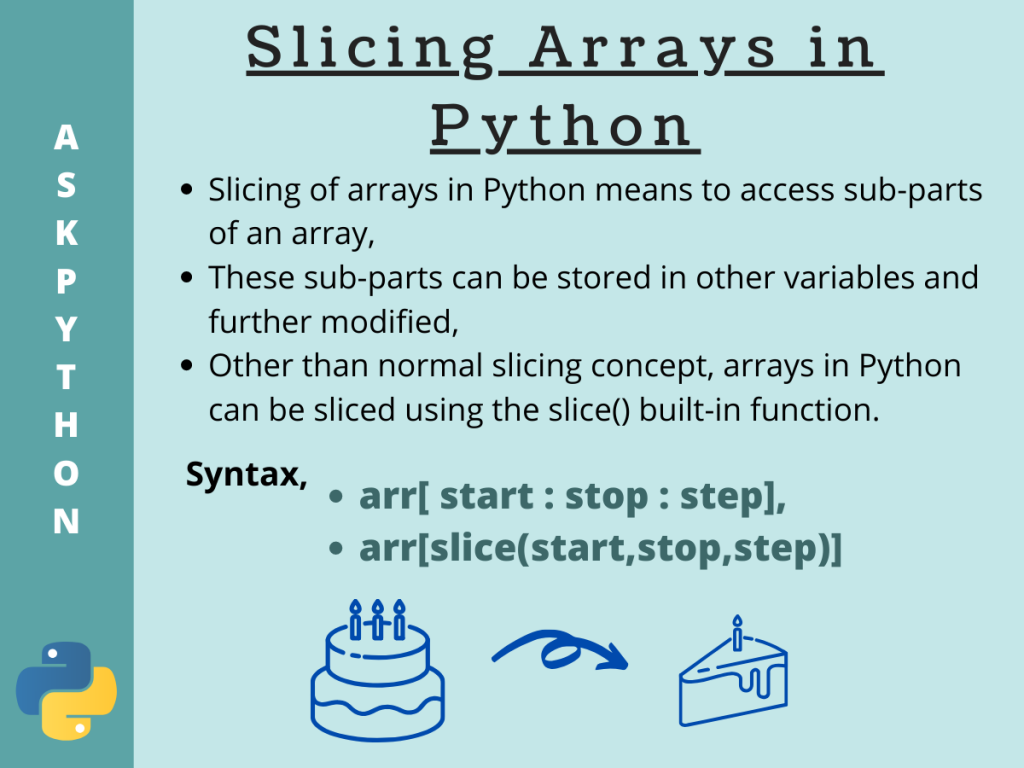# Understanding Array Slicing in PythonArray slicing in Python is a technique in programming that allows you to extract a portion of an array, or a sequence of elements in Python. This technique plays a crucial role in many data manipulation and analysis tasks, and is widely used in various applications ranging from scientific computing, web development, and data analysis.

In this article, you will learn the fundamentals of array slicing in Python, including syntax, examples, and best practices for using this powerful feature in your code.

## What is Array Slicing in Python?

Array slicing in Python is a powerful feature that allows you to create new sub-arrays from existing arrays by specifying the starting and ending indices. The new sub-array is a portion of the original array, and it can be used for a variety of purposes, such as processing specific data, managing memory usage, and more.

Array slicing can be easily done following the Python slicing method. For which the syntax is given below.

```arr[ start : stop : step ]
```

Again, Python also provides a function named slice() which returns a slice object containing the indices to be sliced. The syntax for using this method is given below.

```slice(start, stop[, step])
```

For both the cases,

• start is the starting index from which we need to slice the array arr. By default set to 0,
• stop is the ending index, before which the slicing operation would end. By default equal to the length of the array,
• step is the steps the slicing process would take from start to stop. By default set to 1.

## Methods for Array Slicing in Python

So now that we know the syntax for using both the methods, let us look at some examples and try to understand the slicing procedure.

In the following examples, we are going to consider both arrays from the array module as well as NumPy arrays.

## 1. With one parameter

Default values for start, stop and step are equal to 0, length of the array, and 1 respectively. Hence, specifying either one of the start or stop, we can slice an array.

Let us see how.

```import array
import numpy as np

#array initialisation
array_arr= array.array('i',[1,2,3,4,5])
np_arr = np.array([6,7,8,9,10])

#slicing array with 1 parameter
print("Sliced array: ", array_arr[:3])
print("Sliced NumPy array: ", np_arr[:4])
```

Output:

```Sliced array:  array('i', [1, 2, 3])
Sliced NumPy array:  [6 7 8 9]
```

Here, we have initialized two arrays one from `array` module and another `NumPy` array. Slicing both of them using one parameter results are shown in the output. As we can see for both the cases, start and step are set by default to 0 and 1. The sliced arrays contain elements of indices 0 to (stop-1). This is one of the quickest methods of array slicing in Python.

## 2. Array Slicing in Python With two parameters

Again, specifying any two parameters among the start, stop and end, you can perform array slicing in Python by considering default value for the third parameter.

Let us take an example.

```import array
import numpy as np

#array initialisation
array_arr= array.array('i',[1,2,3,4,5])
np_arr = np.array([6,7,8,9,10])

#slicing array with 2 parameters
print("Sliced array: ", array_arr[2:5])
print("Sliced NumPy array: ", np_arr[1:4])
```

Output:

```Sliced array:  array('i', [3, 4, 5])
Sliced NumPy array:  [7 8 9]
```

In this case, too, the sliced `array` module array and `NumPy` array contain elements of indices specified start to `(stop-1)` with step set to 1. The output is hence justified.

## 3. With the step parameter

When all the three parameters are mentioned, you can perform array slicing in Python from index start to (stop-1) with each index jump equals to the given step.

Look at the example below to have a clear understanding.

```import array
import numpy as np

#array initialisation
array_arr= array.array('i',[1,2,3,4,5,6,7,8,9,10])
np_arr = np.array([11,12,13,14,15,16,17,18,19,20])

#slicing array with step parameter
print("Sliced array: ", array_arr[1:8:2])
print("Sliced NumPy array: ", np_arr[5:9:3])
```

Output:

```Sliced array:  array('i', [2, 4, 6, 8])
Sliced NumPy array:  [16 19]
```

Similarly, here we get sliced arrays with values from the arrays from given indices start to stop-1. The only difference here is the step value, this time it is specified as 2 and 3 for both `array` module array and `NumPy` array respectively. Hence this time each index jump is of the value of the given step.

## 4. Array Slicing in Python with the slice() Method

The `slice()` method in Python returns a sequence of indices ranging from start to stop-1 with the given step value.

Similar to the previous cases, here also the default values of start and stop are 0 and the step is equal to 1.

```import array
import numpy as np

#array initialisation
array_arr = array.array('i',[1,2,3,4,5,6,7,8,9,10])
np_arr = np.array([11,12,13,14,15,16,17,18,19,20])

s = slice(3,9,3)

#slicing array with slice()
print("Sliced array: ", array_arr[s])
print("Sliced NumPy array: ", np_arr[s])
```

Output:

```Sliced array:  array('i', [4, 7])
Sliced NumPy array:  [14 17]
```

Here, firstly we have initialized two arrays, one from `array` module and the other from `NumPy` module. The `slice()` method is called with start, stop and step mentioned as 3, 9 and 3 respectively. Hence, when we pass this sequence `s` to the arrays, we get sliced arrays with values containing the elements at indices 3 and 6.

Hence, the output is justified.

Note: Always the original array is kept intact and stays untouched. If needed, the sliced array can be stored in some variable.

## Conclusion

So in this tutorial, we got to learn about the concept of array slicing in Python. For any further questions feel free to comment it below.